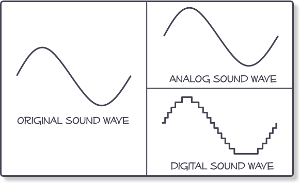# Bit Depth and Sample Rate Explained

When recording audio within a DAW, you will likely be asked what bit depth and sample rate you want to record in.First of all you need an understanding of how digital sound is captured. In this diagram on the digital end you see steps that represent the original wave. Each ‘step’ upwards is a single value, this is the bit depth. These ‘values’ are represented as binary, a 1 or a 0. In this example there are 12 possible values, which can be represented by a 4 bit number.

The sample rate works on the other axis. Sample rate is the number of possible points along the horizontal axis in a second. Unlike bit depth, the sample rate is measured in Hertz. If a second of audio contained 9,000 samples, this would be represented with 4,500Hz.

A standard CD quality track is 44.1kHz and 24 bit. This results in 88,200 samples per second, at a bit depth of 24, meaning 16777215 possible vertical values. Although not identical, as digital sound progresses in quality, we get closer and closer to representing the original analog sound wave.## Akai’s ultra-slim next-gen MPC Studio is here

The new piece of kit features an assignable touch strip and 16 pressure and velocity sensitive pads with aftertouch.

## The Moog Sound Studio now comes as a three-synth package

The Moog Sound Studio includes the Mother -32, DFAM, and Subharmonicon.